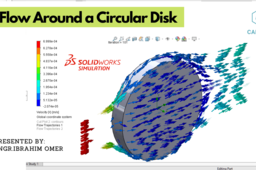# Flow Around a Circular Disc in SOLIDWORKS | Flow Simulation| External Flow |Cylinder Flow SimulationIn this tutorial, we'll learn basic flow simulation in SOLIDWORKS 2020. First of all, we'll model a circular disc and then we'll do a basic simulation. The goal is to find out Drag Force and Drag Coefficient.

Parameters for the study are as follows :

1) Cylinder Diameter=0.02m
2) Cylinder Length=0.03m
3) Dynamic Viscosity=0.0000181 Pa.s
4) Working Medium=Air
5) Reynolds Number=10
6) Velocity=0.0007 m/s
7) Density=1.23 kg/m3
8) Temperature=293.2 K
9) Pressure=101.325 kPa

We will establish drag coefficient equation. We perform simulation, then we will plot results along with graphs and MS word report.

It is a good exercise for beginners and it will be a good practice for beginners. Kindly use play pause technique for practice. I have attached files in the description for practice. Feel free to ask your queries in comment section. I will try to answer each and every question. Keep practicing as practice makes a man perfect.

For Files:
Solidworks Flow Simulaion

#solidworksflo